Vocabulary
Force Fundamentals
Affects of Mass
Predicting Motion
Newton's Laws
100

A push or a pull, which causes movement or change in an object’s movement or shape.

What is a force?

100

This is an example of a force being applied.

What is pulling a wagon?

Answers can vary:  any push or pull.

100This is what Gracie varies in each trial?

What is the mass of the car?

100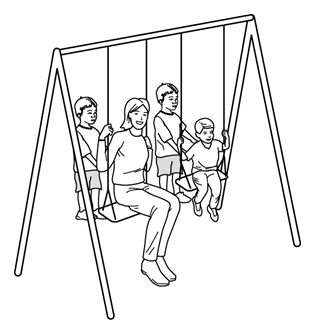This is who will accelerate faster if the two boys use the same force to push a parent and a child.

Who is the child?

100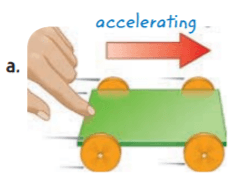This is the type of force causing the cart to accelerate.

What is an unbalanced (push) force?

200

Forces on an object that are equal in size and opposite in direction.

What is a balanced force?

200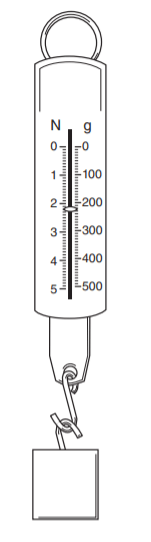This is the force that causes the reading on the spring scale shown in the illustration.

What is gravity?

200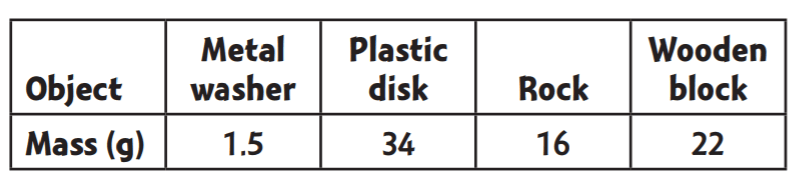This object will require the most force to toss it 2 meters.

What is the plastic disk?

200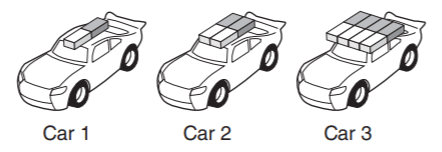This car will travel the least distance if the same amount of force is used to launch each with a rubber band.

What is car 3?

200

This is the force that causes you to stop after you coast down a hill on a bicycle?

What is friction?

From Newton's 3rd Law:  equal and opposite force

300

A force that opposes motion and acts between two objects that are touching.

What is friction?

300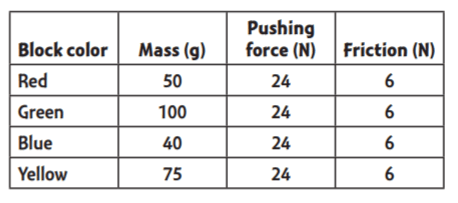According to Newton’s laws of motion, this block will have the greatest change in motion.

What is the blue block?

300

This is what Summer must use if she wants a cart with 10 books on it to go the same distance as the cart that she pushed with 5 books on it.

What is more force?

300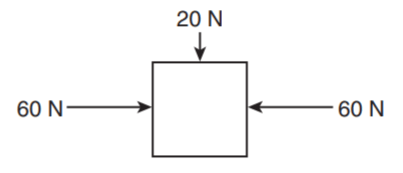This is the direction that the box will travel.

What is down (south)?

300

These are the missing words summarizing Newton’s first law of motion:  Objects at _____ don’t move unless an unbalanced force acts on them, and objects in _______ don’t slow down, speed up, stop, or turn unless a force makes them do so.

What is rest and motion?

400

A force of attraction between two objects, even if they are not touching.

What is gravity?

400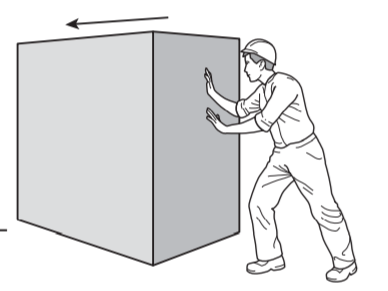This is what keeps the box from moving even though the worker is pushing on it.

What is friction?

400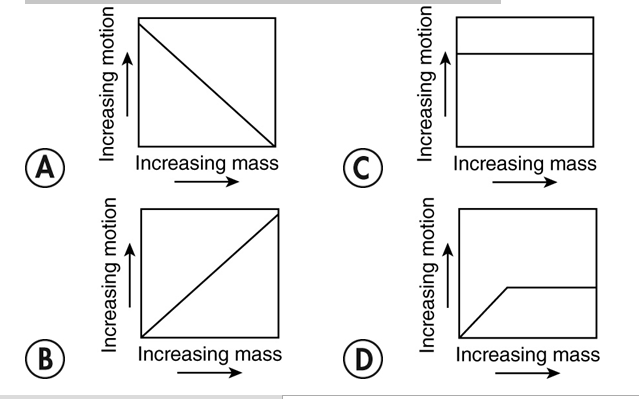This graph shows the relationship between mass and motion when the same force is applied.

What is graph A?

400

This is what will happen to an object traveling in a straight line in space with no forces affecting it.

What is keep moving in the same direction at the same speed?

Inertia

400

These are the two factors that determine an object’s acceleration according to Newton’s second law of motion.

What are the mass of the object and the amount of force applied to the object?

What are mass and force?

500

The behavioral pattern or tendency of objects to resist a change in motion.

What is inertia.

500

This is the reason that a block did not move when Emerson pulled gently on a spring scale even though scale showed a force.

What is the force was balanced by an opposing (opposite force)?

500This is most likely the measurement missing from the table from Karter pushing toy trucks and letting them roll across a flat surface.

What is around 60?  (57)  or

What is a number less that 92 and greater than 30?

500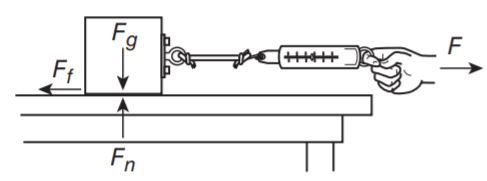When these two forces are equal, the block is not moving.

What are F and Ff?  or

What is the Force of the pull and the Force of friction?

500

Charlotte pushes a bowling ball away from her with a lot of force. Then she repeats the same procedure with a soccer ball. This Newton’s laws explains the difference between the movements of the two balls.

What is Newton's 2nd law?

Mass and Force are inversely related.

Click to zoom